Circles Covering Circles

The following pictures show n unit circles covering the largest known circle (of radius r ).

 n=1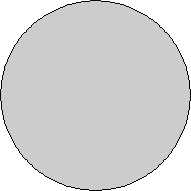r = 1Trivial. n=3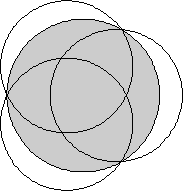r = 2 / √3 = 1.154+Trivial. n=4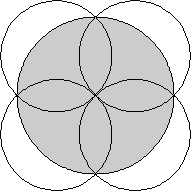r = √2 = 1.414+Trivial. n=5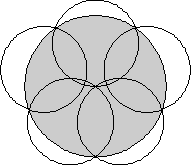r = 1.641+Proved by Károly Bezdekin 1983.

 n=6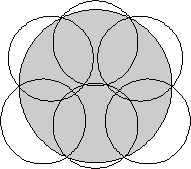r = 1.798+Proved by Károly Bezdekin 1979. n=7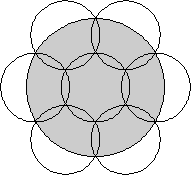r = 2Trivial. n=8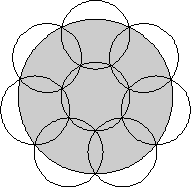r = 2.246+Proved by Gábor Fejes Tóthin 1996. n=9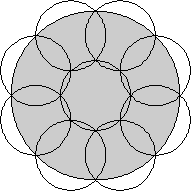r = √2 + 1 = 2.414+Proved by Gábor Fejes Tóthin 1996.

 n=10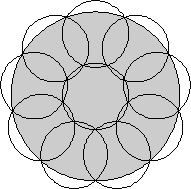r = 2.532+Proved by Gábor Fejes Tóthin 2005. n=11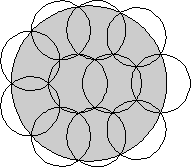r = 2.631+Found by Hans Melissenin 1997. n=12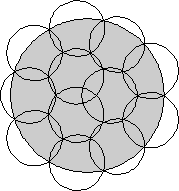r = 2.769+Found by Hans Melissenin 1997. n=13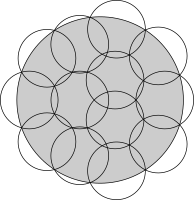r = 2.884+Found by Jeremy Tanin 2018.

 n=14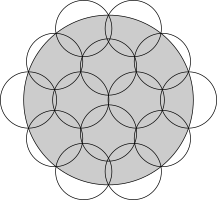r = 3.014+Found by Jeremy Tanin 2018. n=15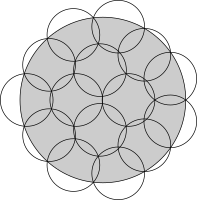r = 3.143+Found by Jeremy Tanin 2018. n=16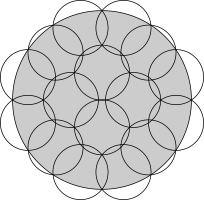r = 3.244+Found by Jeremy Tanin 2018. n=17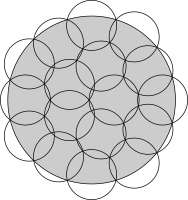r = 3.349+Found by Jeremy Tanin 2018.

 n=18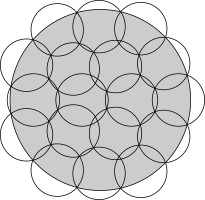r = 3.446+Found by Jeremy Tanin 2018. n=19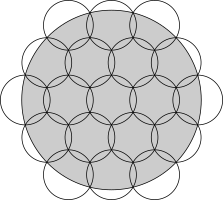r = √13 = 3.605+Found by Jeremy Tanin 2018. n=20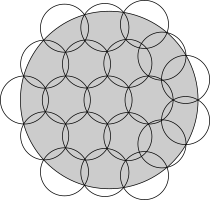r = 3.692+Found by Jeremy Tanin 2018. n=21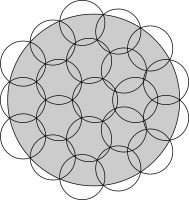r = 3.804+Found by Jeremy Tanin 2018.

 n=22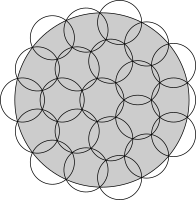r = 3.948+Found by Jeremy Tanin 2018. n=23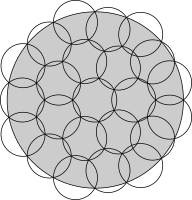r = 4.000+Found by Jeremy Tanin 2018. n=24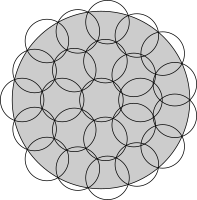r = 4.076+Found by Jeremy Tanin 2018. n=25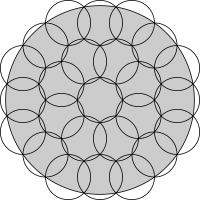r = √6 + √3 = 4.181+Found by Jeremy Tanin 2018.